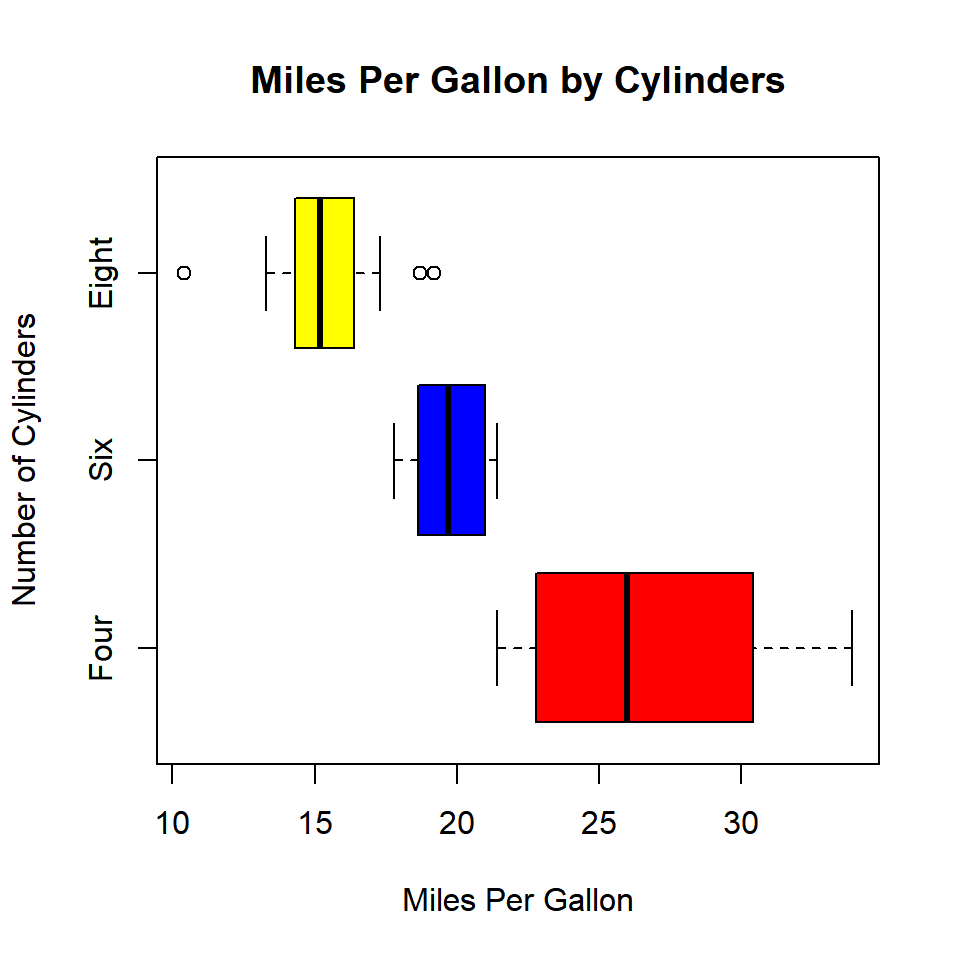# Chapter 6 Box Plots

## 6.1 Introduction

In this chapter, we will learn to

• create univariate/multivariate box plots
• interpret box plots
• create horizontal box plots
• detect outliers
• modify box color
• use formula to compare distributions of different variables
• use notches to compare medians

## 6.2 Box Plot

The box plot is a standardized way of displaying the distribution of data based on the five number summary: minimum, first quartile, median, third quartile, and maximum. Box plots are useful for detecting outliers and for comparing distributions. It shows the shape, central tendancy and variability of the data.

### 6.2.1 Structure

A boxplot splits the data set into quartiles. The body of the boxplot consists of a “box” (hence, the name), which goes from the first quartile (Q1) to the third quartile (Q3). Within the box, a vertical line is drawn at the Q2, the median of the data set. Two horizontal lines, called whiskers, extend from the front and back of the box. The front whisker goes from Q1 to the smallest non-outlier in the data set, and the back whisker goes from Q3 to the largest non-outlier. If the data set includes one or more outliers, they are plotted separately as points on the chart.

## 6.3 Univariate Box Plot

### 6.3.1 Basic Plot

Let us begin by creating a basic box plot. We will use the `boxplot()` function and specify the data.

``boxplot(mtcars\$mpg)``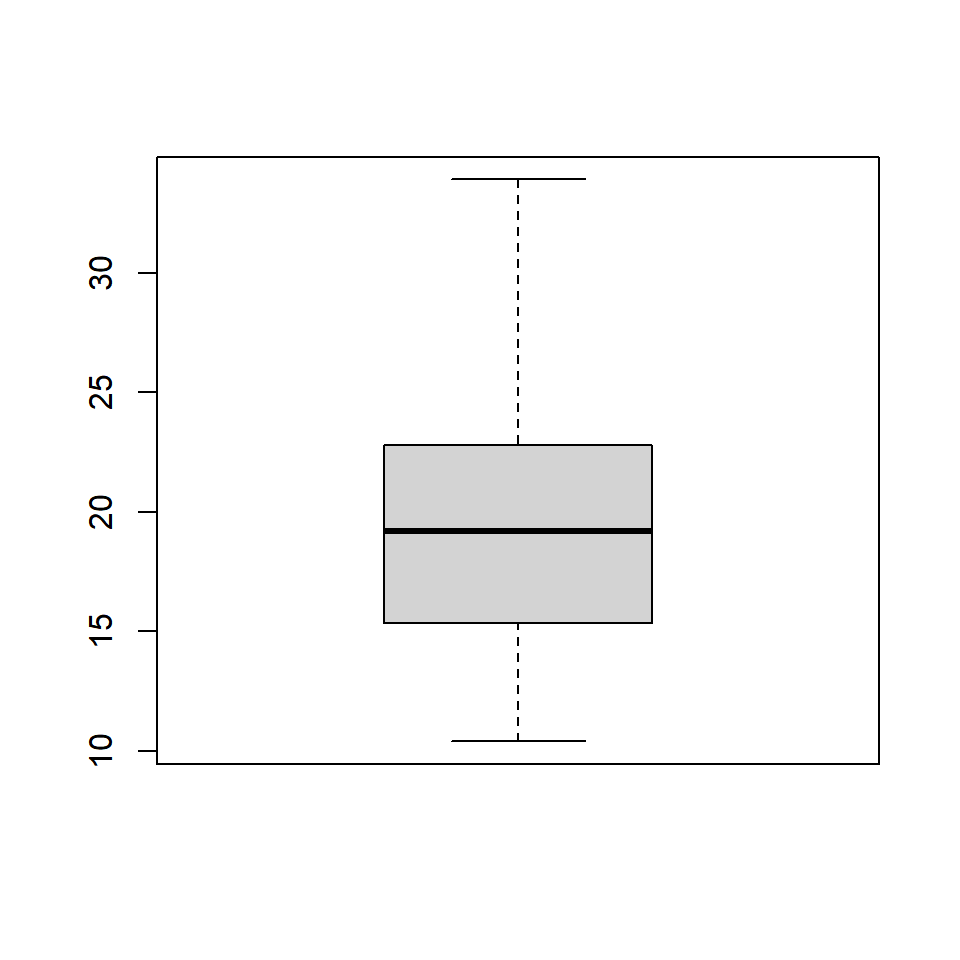### 6.3.2 Horizontal Box Plot

Use the `horizontal` argument in the `boxplot()` function to create a horizontal box plot.

``boxplot(mtcars\$mpg, horizontal = TRUE)``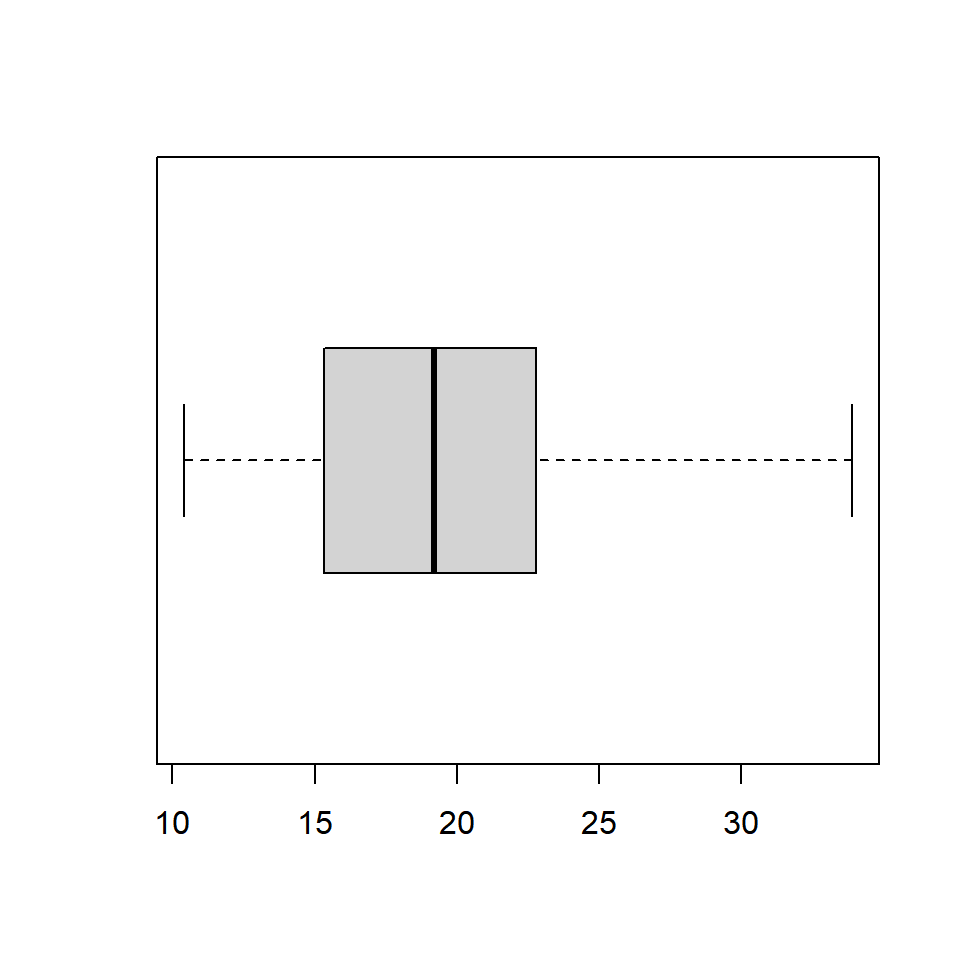### 6.3.3 Color

Let us add some color to the boxplot. Use the `col` argument to specify a color for the plot.

``boxplot(mtcars\$mpg, col = 'blue')``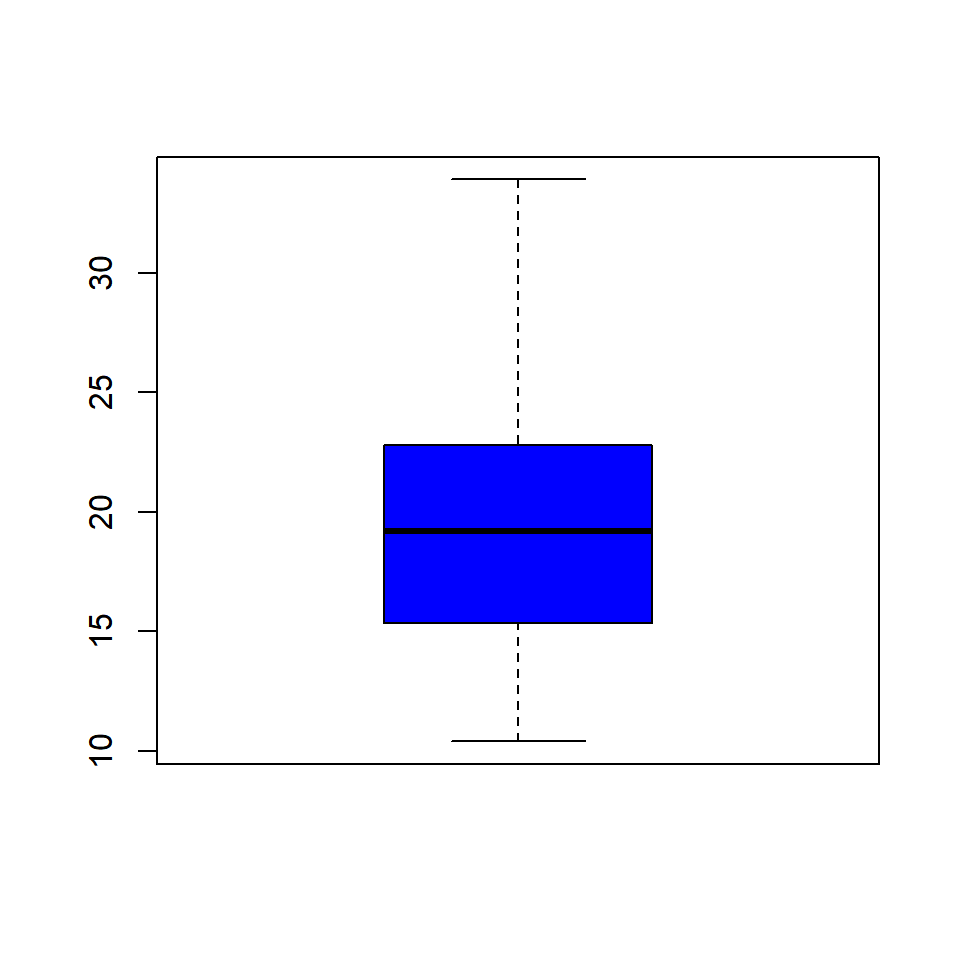### 6.3.4 Border Color

We can specify a separate color for the border of the box in the boxplot. To modify the border color, use the `border` argument.

``boxplot(mtcars\$mpg, border = 'red')``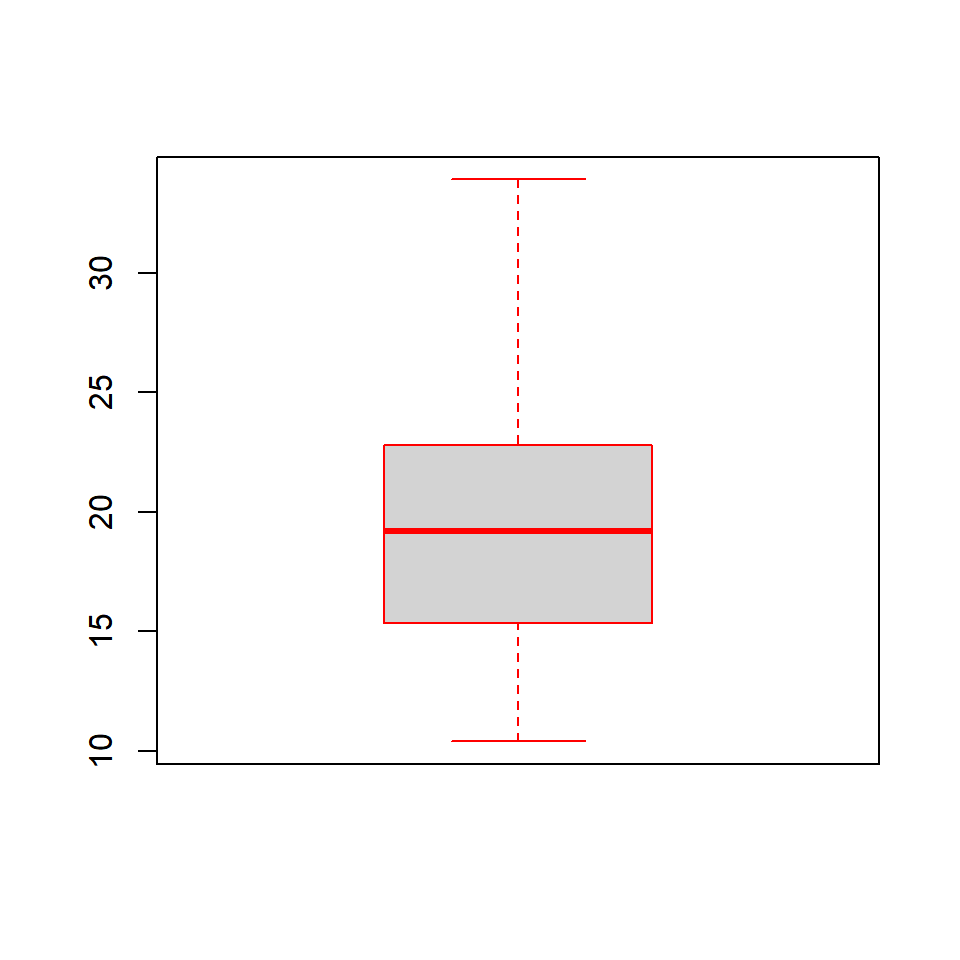### 6.3.5 Range

The `range` argument determines how far the plot whiskers extend out from the box. If range is positive, the whiskers extend to the most extreme data point which is no more than range times the interquartile range from the box. A value of zero causes the whiskers to extend to the data extremes.

Let us set the value of `range` to 0 and observe the plot.

``boxplot(mtcars\$mpg, range = 0)``In the below plot, we set the value of `range` to 1.

``boxplot(mtcars\$mpg, range = 1)``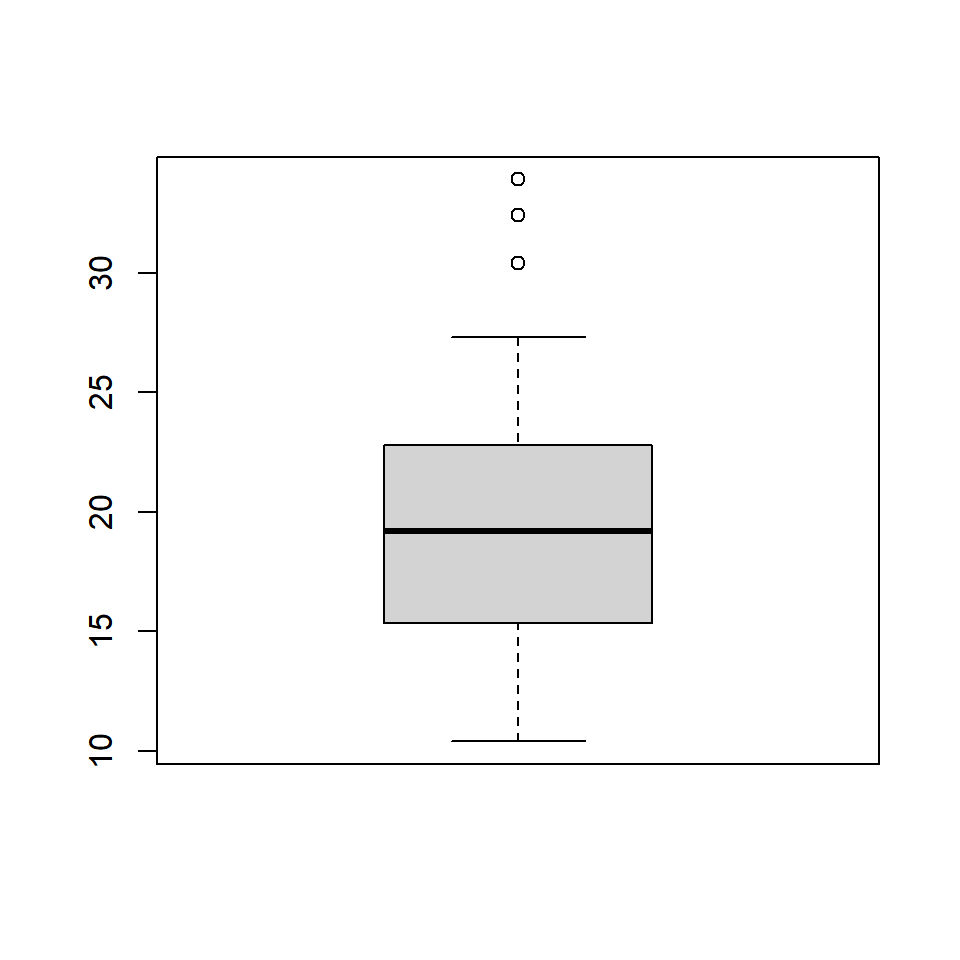Let us observe how the plot appears as we change the value of `range` from 0 to 1.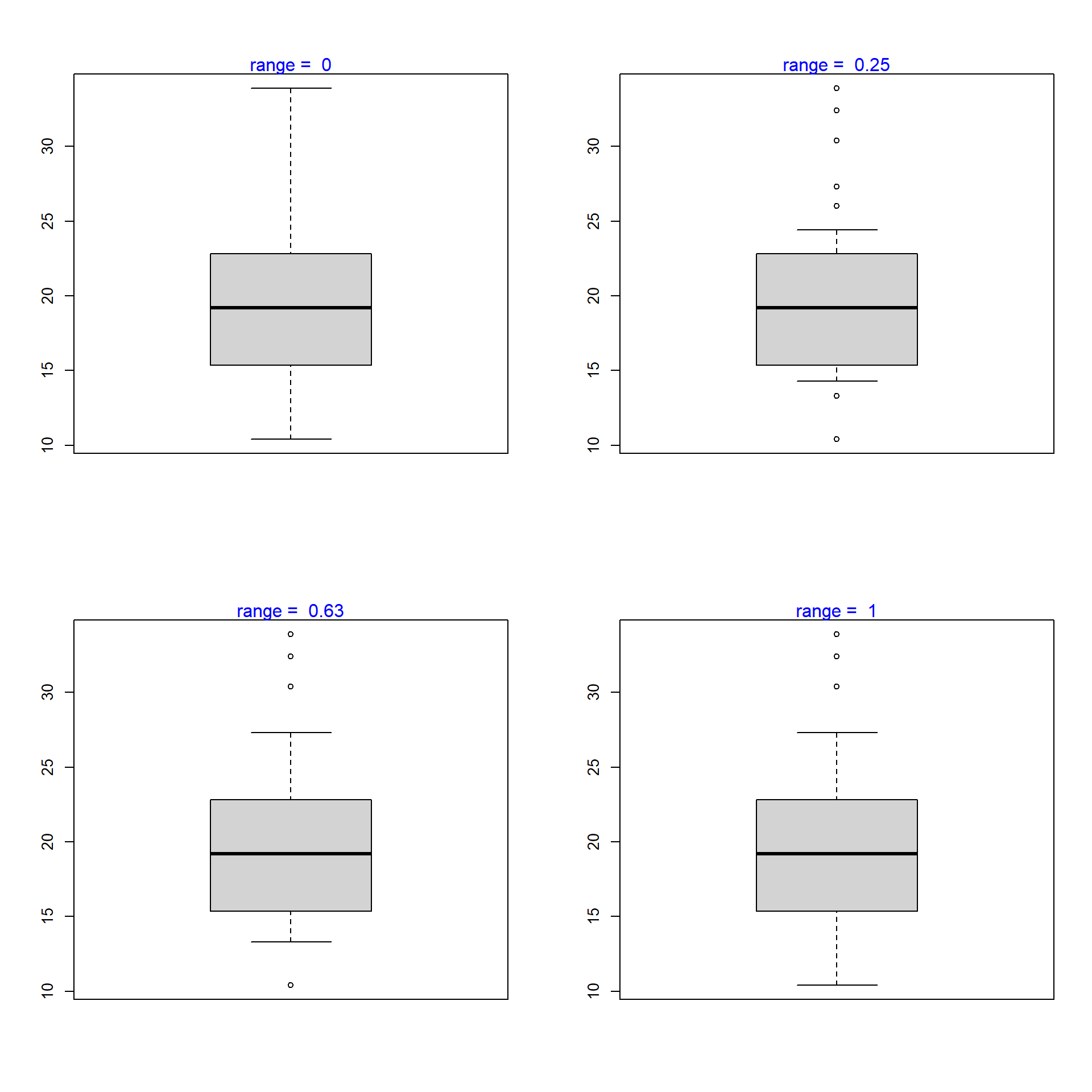### 6.3.6 Outline

The outliers in the plot are not drawn if the `outline` argument is set to `FALSE`. The default value is `TRUE`.

``boxplot(mtcars\$mpg, range = 1, outline = FALSE)``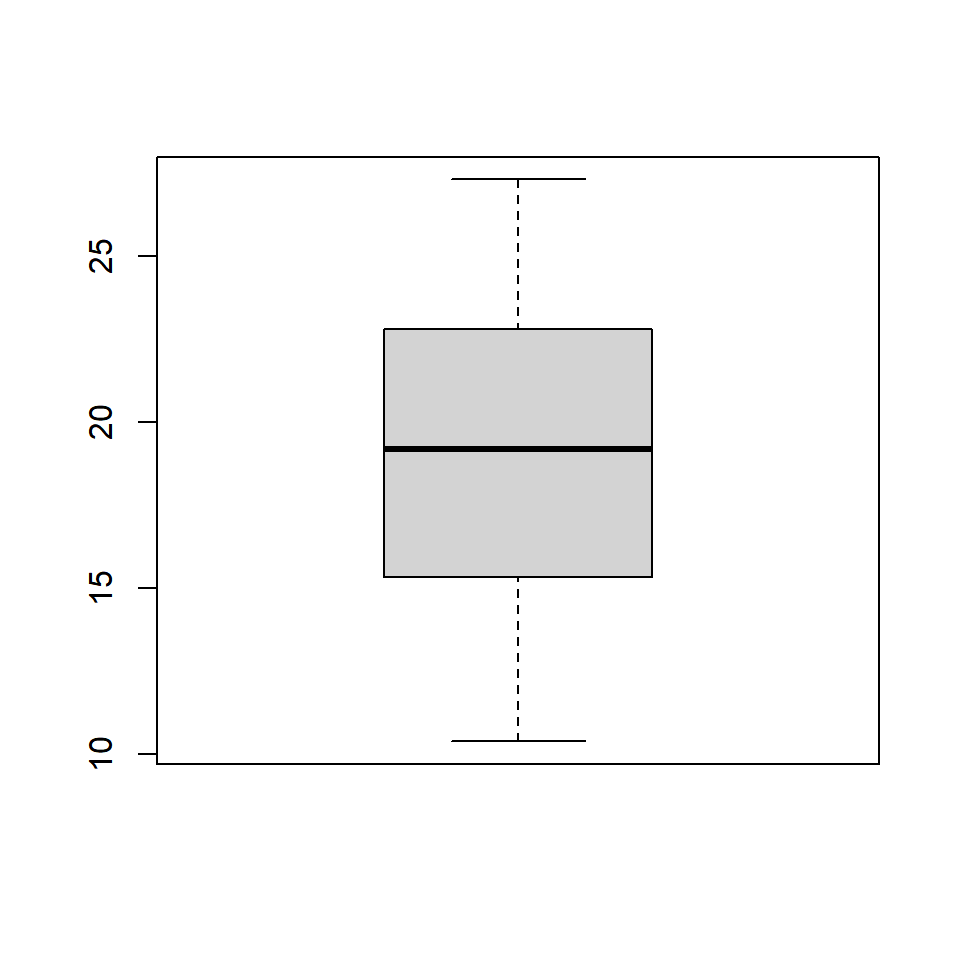The below plot displays how the plot changes with the values set for `outline`: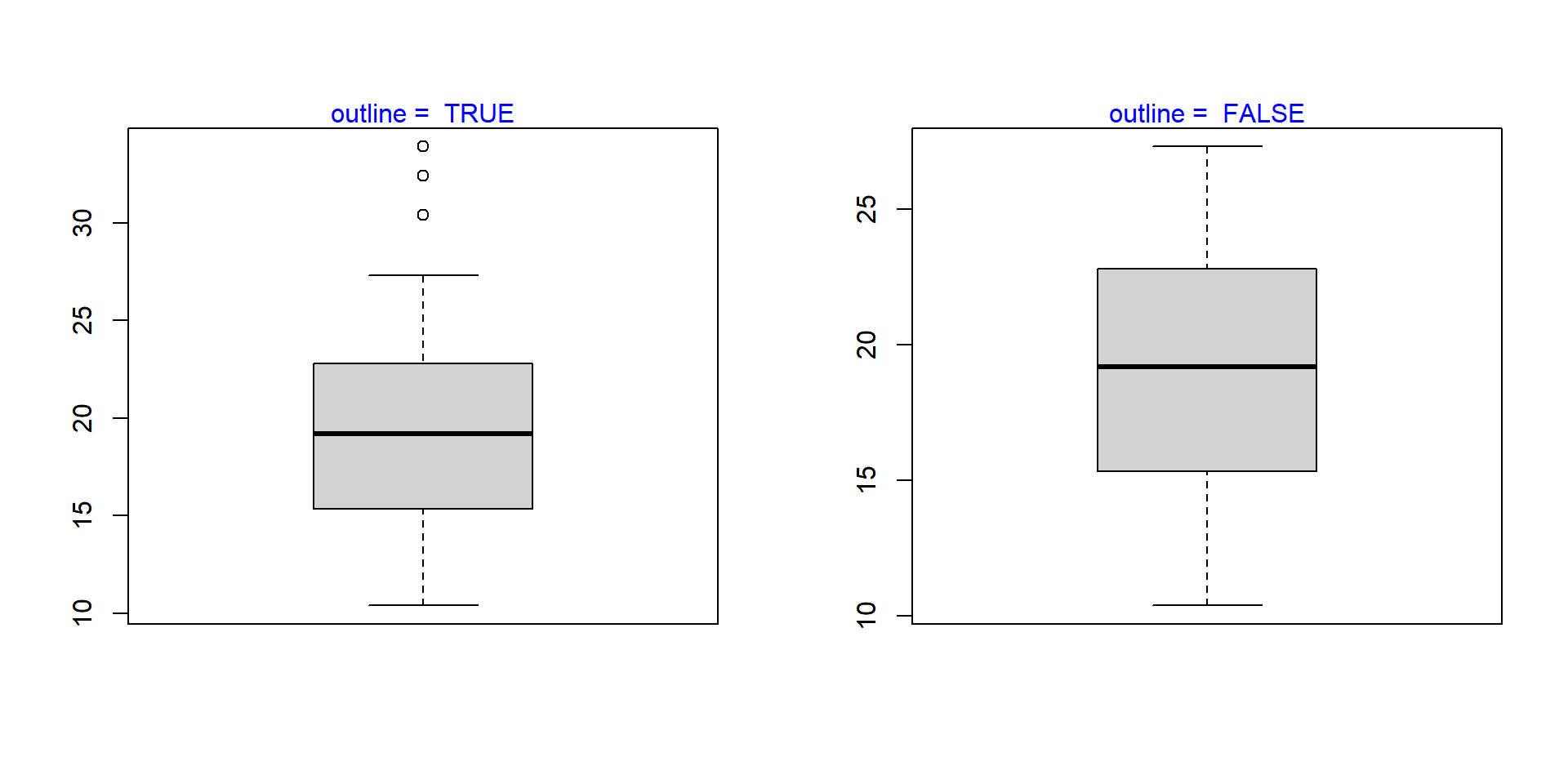### 6.3.7 Varwidth

If the `varwidth` argument is set to `TRUE`, the boxes are drawn with widths proportional to the square-roots of the number of observations in the groups.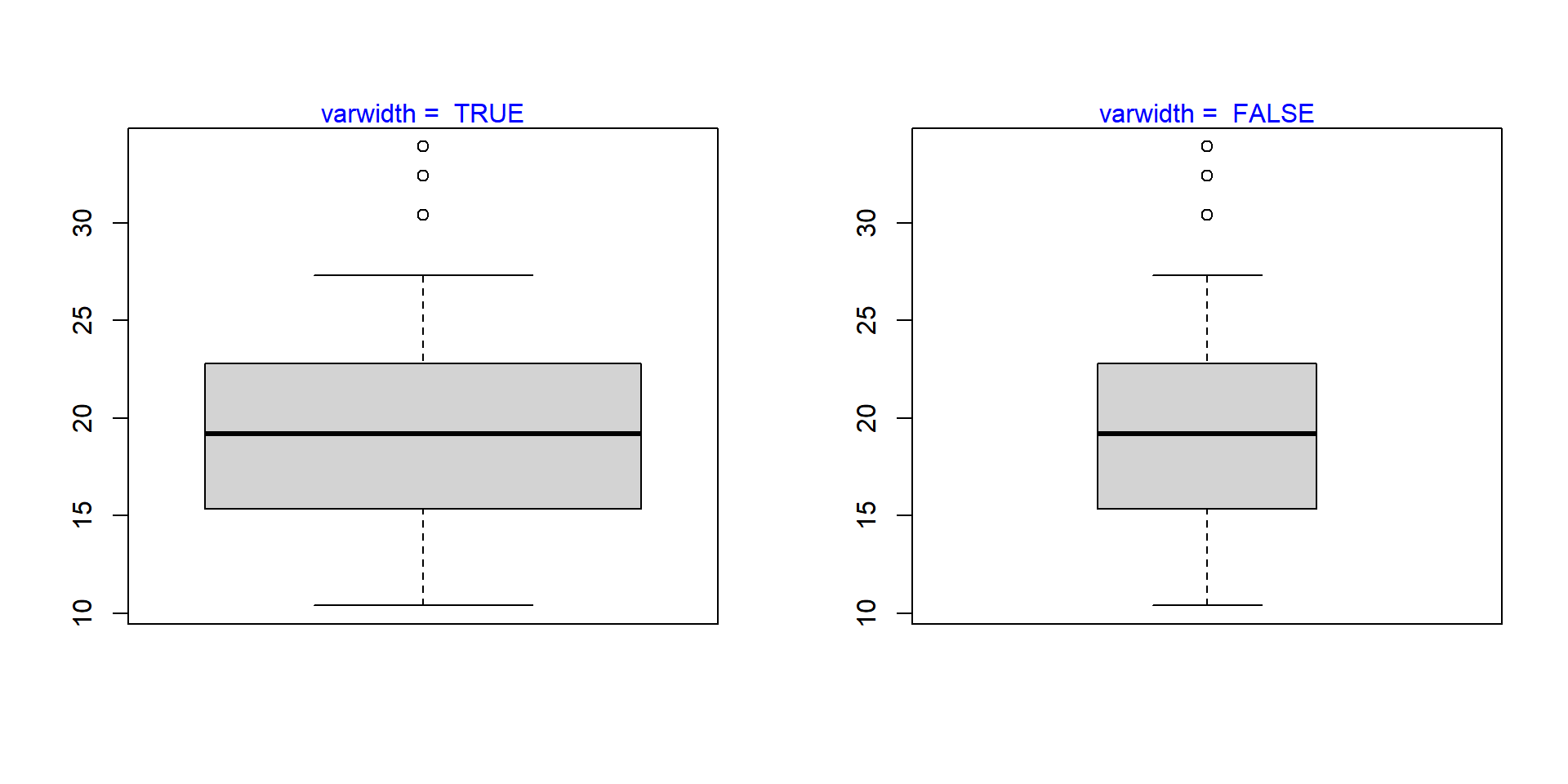## 6.4 Bivariate/Multivariate Box Plot

As we said in the introduction, box plots can be used to compare distributions of several variables. Let us use the `mtcars` data set and compare the distribution of Miles Per Gallon (`mpg`) for automobiles with different number of cylinders (`cyl`). We will do this by specifying a formula as shown in the below example.

``boxplot(mtcars\$mpg ~ mtcars\$cyl)``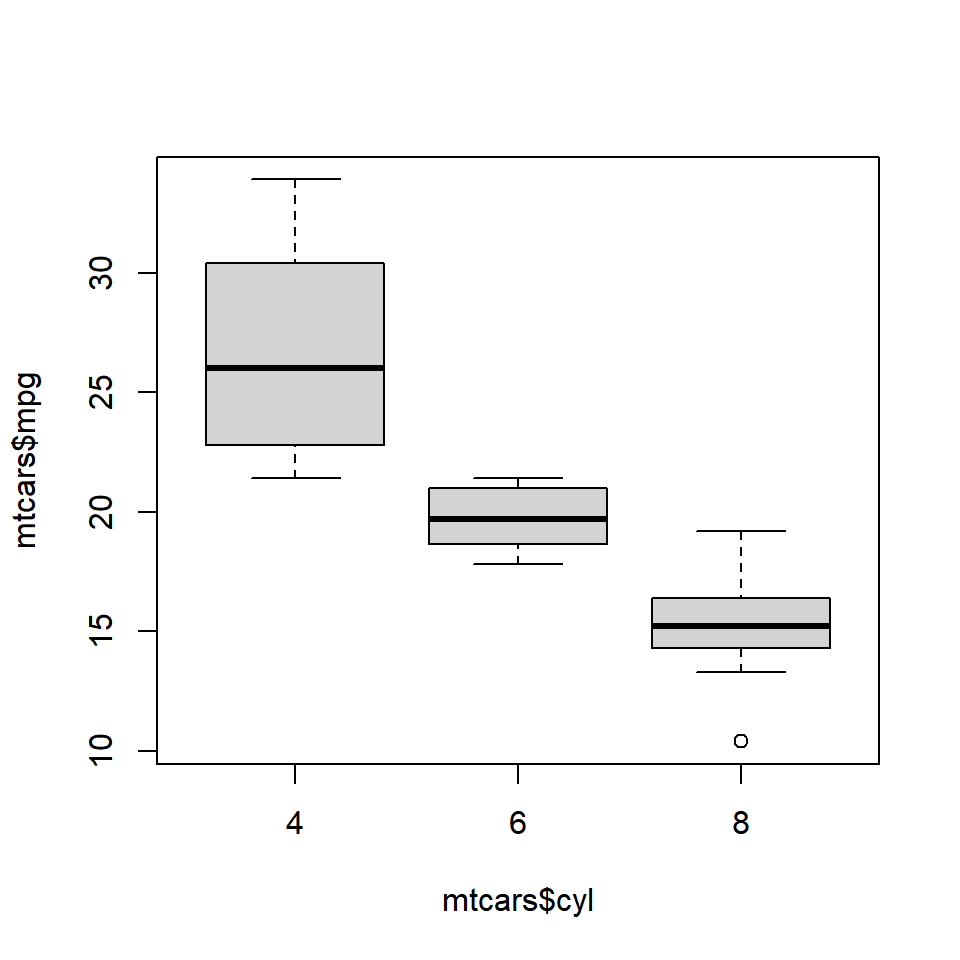We use the formula when we are comparing the distribution of a continuous variable across different levels of a categorical variable. If we want to compare the distributions without using a categorical variable, we need to specify the variable separately in the `boxplot()` function. Below is an illustration of this method. We will split the `mpg` data using the `split()` function and plot them separately. The `split()` function splits a continuous variable based on the levels of a categorical variable.

``````mpg_split <- split(mtcars\$mpg, mtcars\$cyl)

mpg_split
## \$`4`
##   22.8 24.4 22.8 32.4 30.4 33.9 21.5 27.3 26.0 30.4 21.4
##
## \$`6`
##  21.0 21.0 21.4 18.1 19.2 17.8 19.7
##
## \$`8`
##   18.7 14.3 16.4 17.3 15.2 10.4 10.4 14.7 15.5 15.2 13.3 19.2 15.8 15.0

mpg_4 <- mpg_split\$`4`
mpg_6 <- mpg_split\$`6`
mpg_8 <- mpg_split\$`8`

boxplot(mpg_4, mpg_6, mpg_8)``````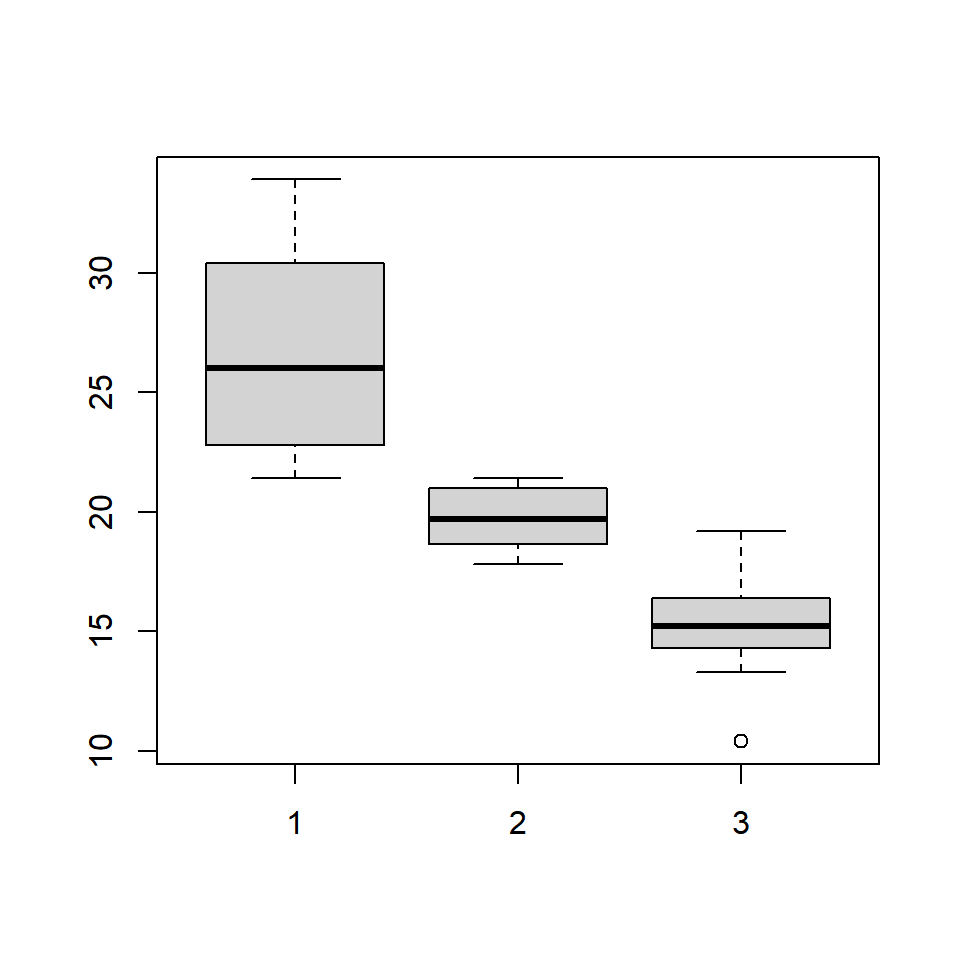The same plot can be created in two ways. If you are comparing the distribution of a continuous variable for the different levels of a categorical variable, use the formula. If you are comparing distribution of independent variables, specify all the variablels in the `boxplot()` function.

### 6.4.1 Color

Let us add some color to the plot. We can specify as many colors as the boxes or we can specify the same color for all of them. Below are two examples where we specify the same color in the first one and different colors in the second one.

#### 6.4.1.1 Single Color

``boxplot(mtcars\$mpg ~ mtcars\$cyl, col = 'blue')``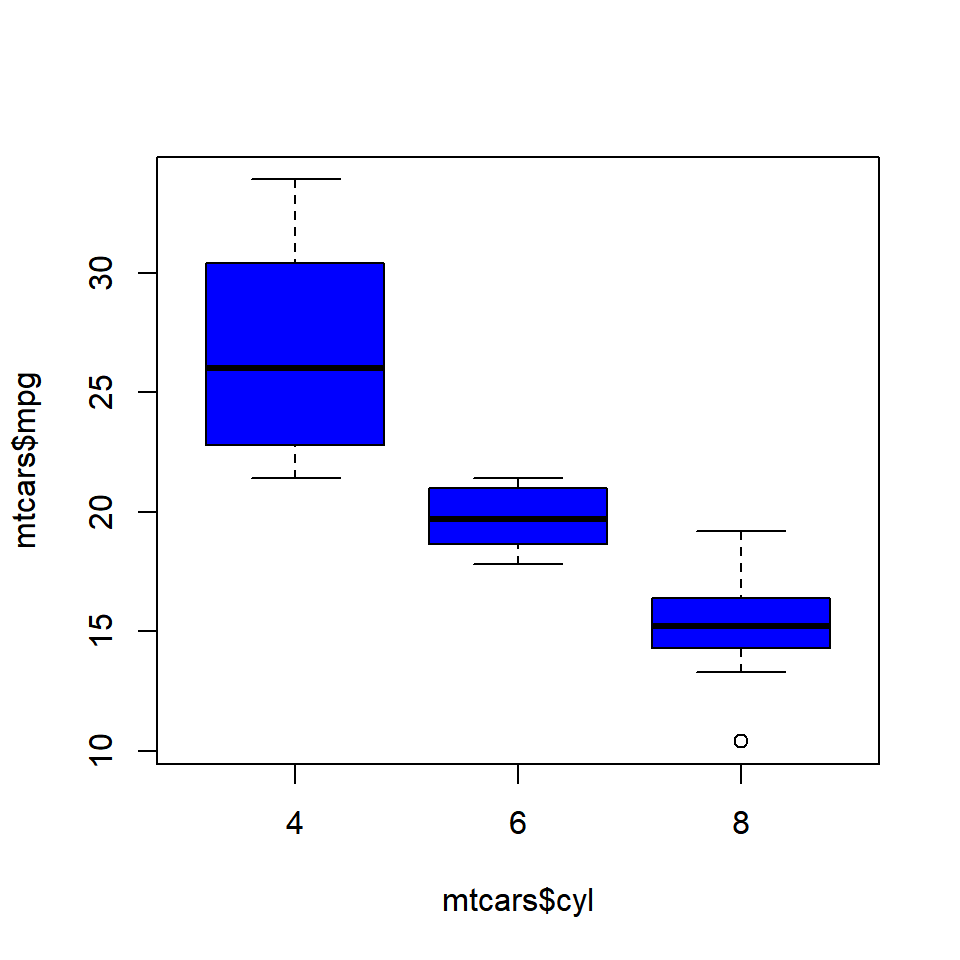#### 6.4.1.2 Different Colors

``````boxplot(mtcars\$mpg ~ mtcars\$cyl,
col = c('red', 'blue', 'yellow'))``````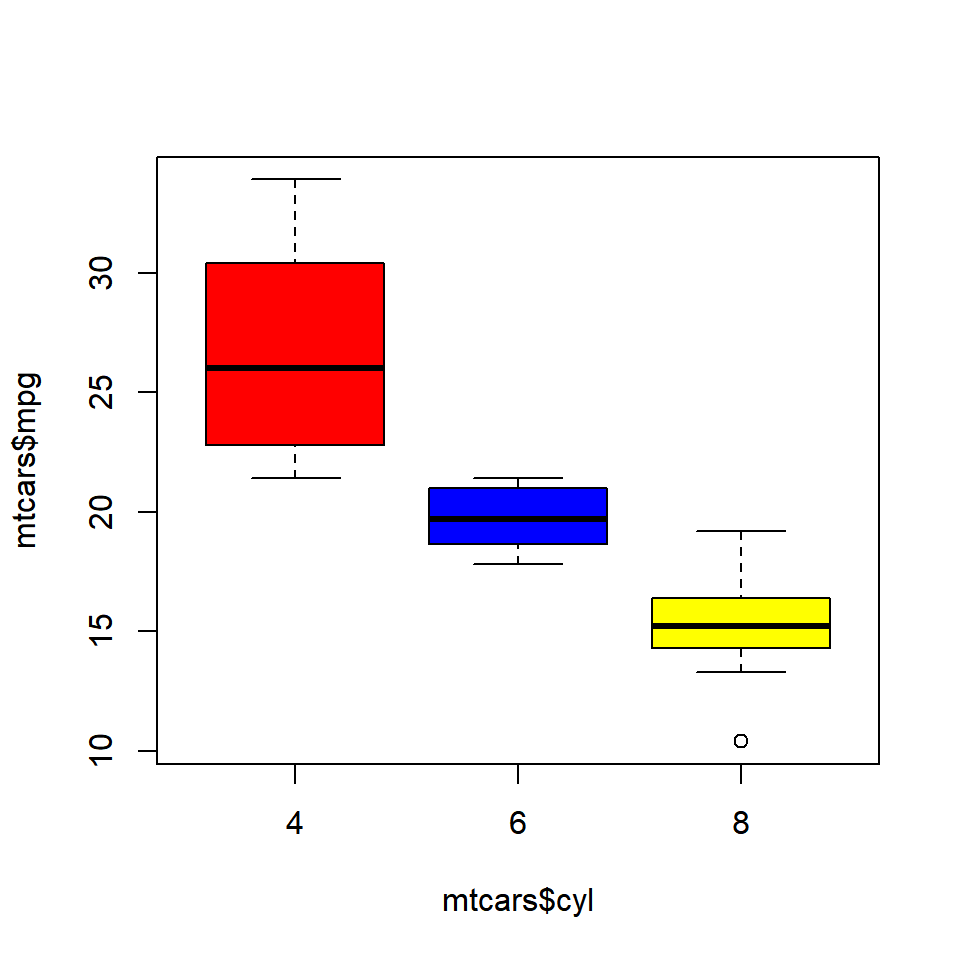### 6.4.2 Compare Medians

If we want to test whether the medians of the different groups differ, we can use the `notch` argument and set it to `TRUE`. A notch is drawn in each side of the boxes and if the notches of the plots do not overlap, it is strong evidence that the medians differ.

We will use a different data set for this example. Download the `hsb2` data from UCLA website and compare the distribution of reading score (`read`) for males and females (`female`).

``````hsb <- read.table('https://stats.idre.ucla.edu/wp-content/uploads/2016/02/hsb2-2.csv', header=T, sep=",")
boxplot(hsb\$read ~ hsb\$female, notch = TRUE,
col = c('red', 'blue'))``````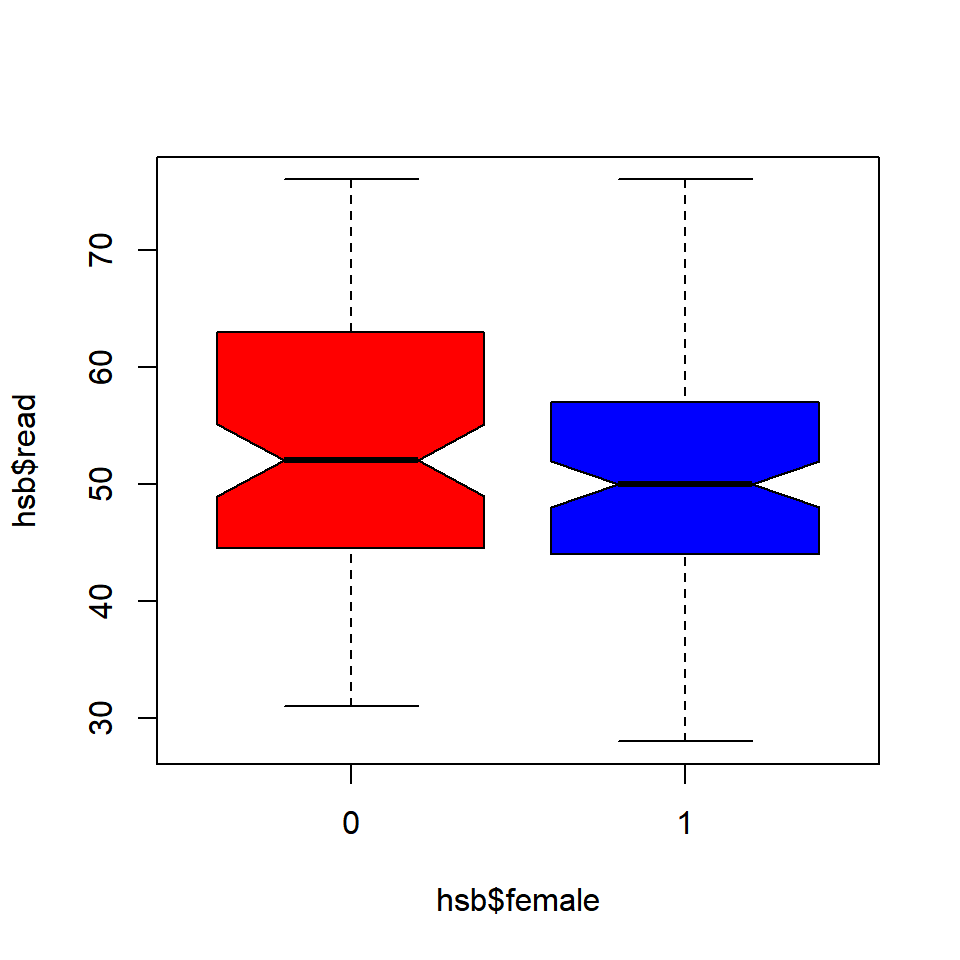Since the notches overlap, there is no strong evidence that the medians differ.

## 6.5 Putting it all together

Let us conclude by adding a title and axis labels to the box plot.

``````boxplot(mtcars\$mpg ~ mtcars\$cyl, range = 1, outline = TRUE,
horizontal = TRUE, col = c('red', 'blue', 'yellow'),
main = 'Miles Per Gallon by Cylinders',
ylab = 'Number of Cylinders', xlab = 'Miles Per Gallon',
names = c('Four', 'Six', 'Eight'))``````